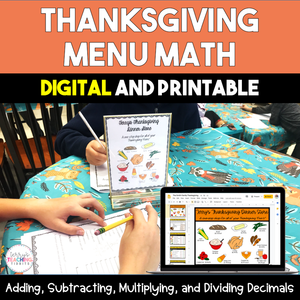• \$6.00
Unit price per
Shipping calculated at checkout.

This Thanksgiving math activity gives students practice with adding, subtracting, multiplying, and dividing decimals. There is a printable version as well as a digital version included (great for remote learning).

Standards:
5.NBT.7 Add, subtract, multiply, divide decimals.
6.NS.3 Fluently add, subtract, multiply, and divide multi-digit decimals using the standard algorithm for each operation.

This Thanksgiving Menu Math activity contains two story problems. Each involves multiplying and dividing decimals. Students will need to calculate the cost of groceries for a Thanksgiving feast. Once they have found the cost of each item, they will need to add them together to find the total cost of groceries. From there, they will need to use division of decimals to find out the cost per person for a Thanksgiving dinner.

The second story problem also contains an enrichment activity in which students will need to apply coupons to the grocery list to find out a revised total cost of groceries, as well as the cost per person.

All digital activities are in Google Slides.

## What You Get

(Printable and Digital)
• introduction mini-lesson (8 slides to introduce sales tax, cost per person, and discounts - great for a formal observation)
• 4 blank restaurant receipts for students to calculate subtotal, tax, and total. This may be used as a partner activity, exit ticket, etc.
• 2 story problems for students to calculate the cost of groceries for a Thanksgiving feast (the story problems included are differentiated for varying levels of students). The second story problem also contains an enrichment activity in which students will need to apply coupons to the grocery list to find out a revised total cost of groceries, as well as the cost per person.